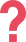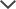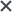Select your pack and start practicing:
I got the TEAS practice tests. Your practice test questions are actually very similar to those in the ‘real’ tests.
Helen
I got the TEAS tests. I found this website to be very helpful. The practice tests are almost identical to the real test; they have helped me a lot in studying.
Chelsea
I used the Wonderlic Basic Skills Test pack. I have nothing to comment. It is perfect.
Bharatkumar
I used the Wonderlic Basic Skills Test pack. The preparation pack was very helpful. It helped me understand the format and style of the WBST which we had never sat before. The explanations after every question were very helpful, as well as the timed tests. A great resource!
Howard
Very good explanations! I love it and I learnt many things from you... thanks for such a great help!
Abigail
Just the timing aspect alone helped me mentally prepare for the time constraint. The other major thing that helped me was the math tips study guide.
Shana
I got the Wonderlic Personality Cognitive prep and it was money well spent!
Maknev

## CLEP Math

Of the 36 test types CLEP offers, four are for math. These tests are Pre-Calculus, Calculus, College Algebra, and College Mathematics. While some of the questions are straightforward math problems, other questions are termed "non-routine" and require you to understand mathematical concepts and apply them correctly in your answers. Furthermore, the different CLEP tests have also a different format. Some tests have a system of two sections whereas others take place a one whole test. For tests with more than one section, a calculator may be allowed for a particular section.

## CLEP for Pre-Calculus

The CLEP pre-calculus test takes a total of 90 minutes to complete and is divided into two sections. The first is a 50-minute section with 25 questions for which you can use a calculator. The second is 40 minutes long, and calculators are not allowed. The test covers the following areas:

• Algebraic expressions, equations, and inequalities
• Functions: concept, properties, and operations
• Representations of functions: symbolic, graphical, and tabular
• Analytic geometry
• Trigonometry and its applications
• Functions as models

The use of an online graphing calculator (non-CAS) is only allowed in the first section of this test. Trigonometry permeates most of the major topics and accounts for more than 15 percent of the exam. The actual proportion of exam questions that requires knowledge of either right triangle trigonometry or the properties of the trigonometric functions is approximately 30–40 percent. Below you will find details about what is expected from each student pertinent to the different topics of the CLEP Pre-Calculus.

Algebraic Expressions, Equations and Inequalities: perform operations on algebraic expressions, solve equations and inequalities (linear, quadratic, absolute value, polynomial, rational, radical, exponential, logarithmic and trigonometric). Ability to solve linear and nonlinear systems of equations.

Functions: Concept, Properties and Operations: demonstrate an understanding of the concept of a function, the general properties of functions (e.g., domain, range), function notation, and the ability to perform symbolic operations with functions (e.g., evaluation, inverse functions).

Representations of Functions: Symbolic, Graphicalperform operations and transformations on functions presented symbolically, graphically or in tabular form. In addition to this, the student is expected to recognize and show an understanding of basic properties of functions and elementary functions (linear, quadratic, absolute value, square root, polynomial, rational, exponential, logarithmic, trigonometric, inverse trigonometric and piecewise-defined functions) that are presented in the forms above.

Analytic Geometry: understand the analytic geometry of lines, circles, parabolas, ellipses and hyperbolas.

Trigonometry and Its Applications: understand basic trigonometric functions, their inverses and apply basic trigonometric ratios and identities (in right triangles and on the unit circle). Moreover, the student has to know how to apply trigonometry in various problem-solving contexts.

Functions as Models: interpret and construct functions as models and to translate ideas among symbolic, graphical, tabular and verbal representations of functions.

## CLEP for Calculus

The calculus CLEP test is a 90-minute test divided into two sections. Section 1 is 50 minutes long and includes 27 questions for which you cannot use a calculator. Section 2 is 40 minutes in length, with 17 questions and the use of calculators permitted. The topics on the calculus test include limits, integral calculus, and differential calculus. About half of the questions on the test involve routine calculus problems, while the other half are "non-routine".

Some of the topics surrounding the CLEP Calculus test are: integral and differential calculus (this includes finding the derivative or integral of elementary functions, understanding related theorems and definitions, etc.) and their properties, limits (calculating, algebra of limits, related theorems, etc.).

## CLEP for College Algebra

The algebra test for CLEP is also a 90-minute test with 60 questions to be answered in that time frame. You are allowed to use a calculator for the entire test. The test is broken down as follows:

• 15 questions on algebraic operations
• 15 questions on equations and inequalities
• 18 questions on functions and their properties
• 12 questions on number systems and operations

The algebra questions are also a mix of routine and non-routine questions. Each test may contain a variety of functions, including linear, polynomial (degree smaller or equal to five), rational, absolute value, power, exponential, logarithmic and piece-wise defined. Below you will find a breakdown of the CLEP Algebra topics.

Algebraic Operations: Polynomials (including factoring and expanding), operations with exponents and algebraic expressions in general, radical functions, logarithmic functions and more should be clear and in full control.

Equations and Inequalities: The student should be able to solve both equations and inequalities with linear and quadratic functions, absolute value, and systems of equations. In addition, exponential and logarithmic equations are also expected in full mastery.

Functions and their properties: Domain and range, algebra of functions, graphs and their properties (including intercepts, symmetry, and transformations), inverse functions.

Number Systems and Operations: Real numbers, complex numbers, sequences and series, factorials and binomial theorem, determinants of 2-by-2 matrices.

## Preparing for CLEP Math Tests

Only students with passing scores on the CLEP tests receive the college credit which means you need to be fully prepared for test day. Reviewing the concepts and working through practice questions can help ensure that test day passes without a hitch.

Making sure you are ready to ace your test is TestPrep-Online's mission. Our practice tests are specifically designed to simulate the real exam and give you all the practice you need. With the help of our detailed answer explanations and study guide, you'll be able to pass the CLEP math test with confidence and ease!

CLEP and other trademarks are the property of their respective trademark holders. None of the trademark holders are affiliated with TestPrep-online or this website.
Reviews

Need HelpNeed Help